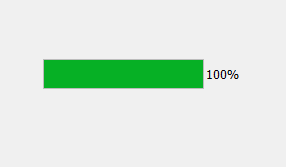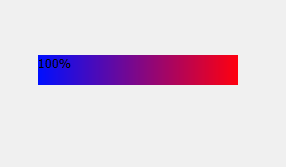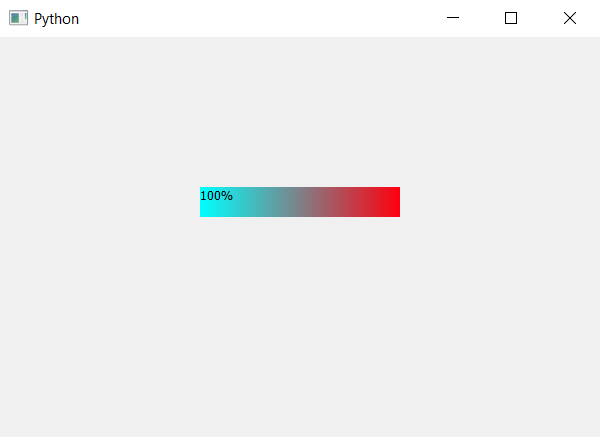Open In App

# PyQt5 – Gradient color Bar of Progress Bar

In this article we will see how we can create the gradient color to bar of progress bar. Gradient Color : In computer graphics, a color gradient specifies a range of position-dependent colors, usually used to fill a region. For example, many window managers allow the screen background to be specified as a gradient. The colors produced by a gradient vary continuously with position, producing smooth color transitions. Below is how normal bar of progress bar looks vs how gradient color bar of progress bar looks:Style sheet code :

```QProgressBar::chunk
{
background: QLinearGradient( x1: 0, y1: 0,
x2: 1, y2: 0,
stop: 0 #000fff,
stop: 1 #ff000f );
}```

This style sheet is used with the help of setStyleSheet method.

## Python3

 `# importing libraries``from` `PyQt5.QtWidgets ``import` `*``from` `PyQt5 ``import` `QtCore, QtGui``from` `PyQt5.QtGui ``import` `*``from` `PyQt5.QtCore ``import` `*``import` `sys`  `class` `Window(QMainWindow):`  `    ``def` `__init__(``self``):``        ``super``().__init__()` `        ``# setting title``        ``self``.setWindowTitle("Python ")` `        ``# setting geometry``        ``self``.setGeometry(``100``, ``100``, ``600``, ``400``)` `        ``# calling method``        ``self``.UiComponents()` `        ``# showing all the widgets``        ``self``.show()` `    ``# method for widgets``    ``def` `UiComponents(``self``):` `        ``# creating progress bar``        ``bar ``=` `QProgressBar(``self``)` `        ``# setting geometry to progress bar``        ``bar.setGeometry(``200``, ``150``, ``200``, ``30``)` `        ``# set value to progress bar``        ``bar.setValue(``100``)` `        ``# setting gradient color to bar``        ``bar.setStyleSheet("QProgressBar::chunk "``                          ``"{"``                          ``"background: QLinearGradient( x1: ``0``, y1: ``0``,``                                                        ``x2: ``1``, y2: ``0``,``                                                    ``stop: ``0` `# 00ffff,``                                                   ``stop: ``1` `# ff000f );"``                          ``"}")` `# create pyqt5 app``App ``=` `QApplication(sys.argv)` `# create the instance of our Window``window ``=` `Window()` `# start the app``sys.exit(App.``exec``())`

Output :